## Flip Flops-

Before you go through this article, make sure that you have gone through the previous article on Flip Flops.

We have discussed-

• A Flip Flop is a memory element that is capable of storing one bit of information.
• It is also called as Bistable Multivibrator since it has two stable states either 0 or 1.

There are following 4 basic types of flip flops-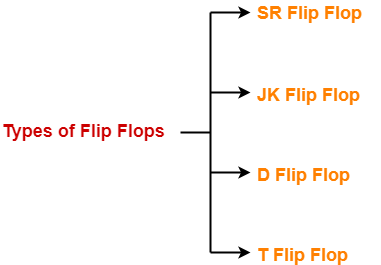1. SR Flip Flop
2. JK Flip Flop
3. D Flip Flop
4. T Flip Flop

## SR Flip Flop-

• SR flip flop is the simplest type of flip flops.
• It stands for Set Reset flip flop.
• It is a clocked flip flop.

## Construction of SR Flip Flop-

There are following two methods for constructing a SR flip flop-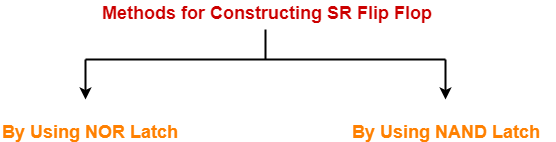1. By using NOR latch
2. By using NAND latch

### 1. Construction of SR Flip Flop By Using NOR Latch-

This method of constructing SR Flip Flop uses-

• NOR latch
• Two AND gates

### Logic Circuit-

The logic circuit for SR Flip Flop constructed using NOR latch is as shown below-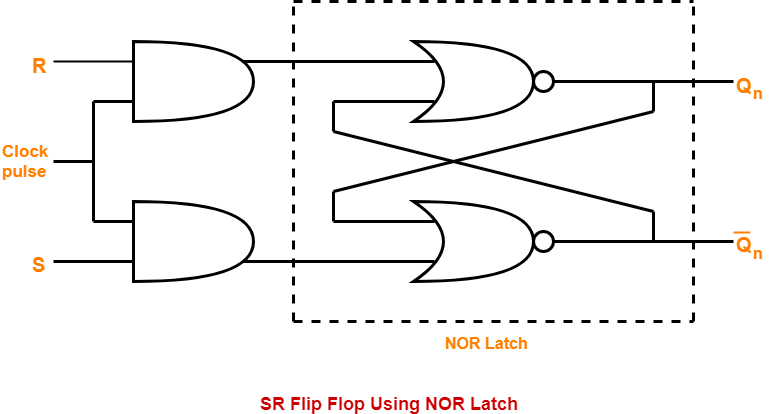### 2. Construction of SR Flip Flop By Using NAND Latch-

This method of constructing SR Flip Flop uses-

• NAND latch
• Two NAND gates

### Logic Circuit-

The logic circuit for SR Flip Flop constructed using NAND latch is as shown below-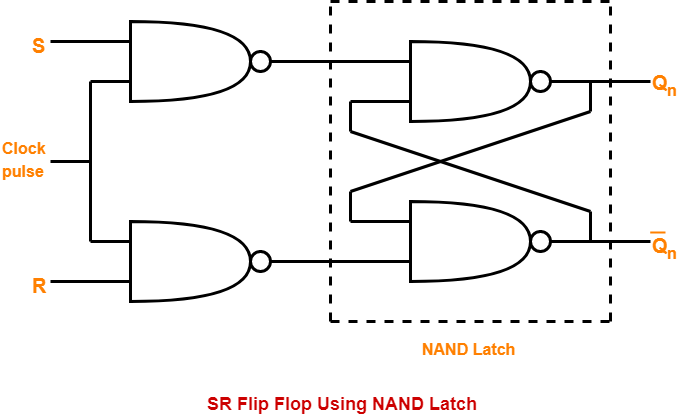### Logic Symbol-

The logic symbol for SR Flip Flop is as shown below-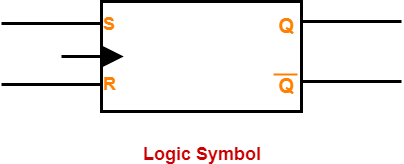### Truth Table-

The truth table for SR Flip Flop is as shown below-

 INPUTS OUTPUTS S R Qn (Present State) Qn+1 (Next State) 0 0 0 0 0 0 1 1 0 1 0 0 0 1 1 0 1 0 0 1 1 0 1 1 1 1 0 Indeterminate 1 1 1 Indeterminate

### Truth Table

The above truth table may be reduced as-

 INPUTS OUTPUTS REMARKS S R Qn (Present State) Qn+1 (Next State) States and Conditions 0 0 X Qn Hold State condition S = R = 0 0 1 X 0 Reset state condition S = 0 , R = 1 1 0 X 1 Set state condition S = 1 , R = 0 1 1 X Indeterminate Indeterminate state condition S = R = 1

### Characteristic Equation-

Draw a k map using the above truth table-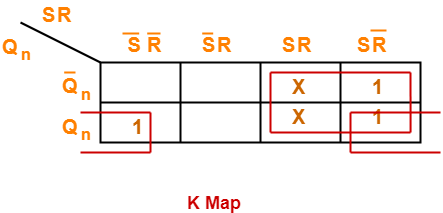From here-

Qn+1 = ( SR + SR’ ) ( Qn +  Q’n ) + Qn ( S’R’ + SR’ )

 Qn+1 = S + QnR’

### Excitation Table-

The excitation table of any flip flop is drawn using its truth table.

 What is excitation table?For a given combination of present state Qn and next state Qn+1, excitation table tell the inputs required.

 Qn Qn+1 S R 0 0 0 X 0 1 1 0 1 0 0 1 1 1 X 0

### Excitation Table

To gain better understanding about SR Flip Flop,

Watch this Video Lecture

Next Article- JK Flip Flop

Get more notes and other study material of Digital Design.

Watch video lectures by visiting our YouTube channel LearnVidFun.